# Plus One Physics Solution Chapter3 Motion in a Straight Line

We are providing a complete and detailed textbook Solution for  Chapter 3 Motion in a Straight Line.Spend more time learning and less time trying to understand complicated physics problems. We’re called physics textbook solution hss plus one for a reason.

Physics plays an important role in our daily life so it’s also an essential subject to study particularly because it opens the gateway to engineering and technical fields like aviation, civil engineering, aeronautical engineering, mechanical engineering, etc.  So after studying this chapter thoroughly you will acquire more understanding of the physical world that surrounds us.

 Board SCERT, Kerala Text Book NCERT Based Class Plus One Subject Physics Textbook Solution Chapter Chapter 3 Chapter Name Motion in a Straight Line Category Plus One Kerala

## Kerala Syllabus Plus One Physics Textbook Solution Chapter 3 Motion in a Straight Line

### Chapter 3 Motion in a Straight Line

Question 1.
In which of the following examples of motion, can the body be considered approximately a point object:
(a) a railway carriage moving without jerks between two stations.
(b) a monkey sitting on top of a man cycling smoothly on a circular track.
(c) a spinning cricket ball that turns sharply on hitting the ground.
(d) a tumbling beaker that has slipped off the edge of a table.
(a) The carriage can be considered a point object because the distance between two stations is very large as compared to the size of the railway carriage.

(b) The monkey can be considered as a point object if the cyclist describes a circular track of very large radius because in that case the distance covered by the cyclist is quite large as compared to the size of monkey. The monkey can not be considered as a point object if the cyclist describes a circular track of small radius because in that case the distance covered by the cyclist is not very large as compared to the size of the monkey.

(c) The spinning cricket ball can not be considered as a point object because the size of the spinning cricket ball is quite appreciable as compared to the distance through which the ball may turn on hitting the ground.

(d) A beaker slipping off the edge of a table can not be considered as a point object because the size of the beaker is not negligible as compared to the height of the table.

Question 2.
The position-time (x-t) graphs for two children A and B returning from their school O to their homes P and Q respectively are shown in figure. Choose the correct entries in the brackets below:(a) (A/B) lives closer to the school than (B/A)
(b) (A/B) starts from the school earlier than (B/A)
(c) (A/B) walks faster than (B/A)
(d) A and B reach home at the (same/different) time
(e) (A/B) overtakes (B/A) on the road (once/ twice).
(a) It is clear from the graph that OQ > OP, so A lives closer to the school than B.

(b) The position-time (x-t) graph of A starts from the origin, so x = 0, t = 0 for A while the (x-t) graph of B starts from C which shows that B starts later than A after a time interval OC. So A starts from school (O) earlier than B.(c) The speed is represented by the slope or steepness of the (x-t) graph. More steeper the graph, more will be the speed, so faster will be the child having steeper graph. As the (x-t) graph of B is steeper than the (x-t) graph of A, so we conclude that B walks faster than

(d) Corresponding to P and Q, the value of t from (x-t) graphs for A and B is same i.e. OE. So both A and B reach home at the same time.

(e) As the (x-t) graphs for A and B intersect each other at one point i.e. D and B starts from the school later, so B overtakes A on the road only once.

Question 3.
A woman starts from her home at 9.00 am, walks with a speed of 5 km h-1 on a straight road up to her office 2.5 km away, stays at the office up to 5.00 pm, and returns home by an auto with a speed of 25 km h-1. Choose suitable scales and plot the (x-t) graph of her motion.
From home to office: Time at which she leaves home for the office 9 am.
speed = 5 km h-1; distance = 2.5 km
Therefore, time taken to reach the office,Thus, time at which she reaches her office = 9.30 am.
Between 9.30 am to 5 pm, she remains in her office i.e. at a distance of 2.5 km from her

From office to home : Time at which she leaves her office = 5 pm.
Speed = 25 km h-1, distance = 2.5 km
Therefore, time taken to reach the home,Therefore, time at which she reaches her home = 5.06 pm.
Hence, (x-t) graph of her motion will be as shown in figure.Question 4.
A drunkard walking in a narrow lane takes 5 steps forward and 3 steps backward, followed again by 5 steps forward and 3 steps backward, and so on. Each step is 1 m long and requires 1 s. Plot the x-t graph of his motion. Determine graphically and otherwise how long the drunkard takes to fall in a pit 13 m away from the start.
The x-t graph of drunkard is shown in figure.Length of each step = 1 m, time taken for each step = 1 s.
∴ Time taken to move 5 steps = 5 s.
5 steps (i.e. 5 m) forward and 3 steps (i.e. 3 m) backward means that the net distance covered by him in first 8 steps i.e. in 8s = 5m-3m = 2 m.
Distance covered by him in first 16 steps or 16s = 2 + 2 = 4m.
Distance covered by the drunkard in first 24 i.e. 24 steps = 2 + 2 + 2 = 6m and distance covered in 32 steps
i.e. 32 sec = 8 m.
∴ Distance covered in first 37 steps = 8 + 5 = 13 m.
Distance of the pit from the start = 13 m.
Total time taken by the drunkard to fall in the pit = 37 s.
Since 1 step requires 1 s of time, so we arrive at  the same result from the graph shown.

Question 5.
A jet airplane travelling at the speed of 500 km h-1 ejects its products of combustion at the speed of 1500 km h-1 relative to the jet plane. What is the speed of the latter with respect to an observer on the ground?
Let υj, υg and υo be the velocities of jet, ejected gases i.e. combustion products and observer on the ground respectively.
Let jet be moving towards right (+ve direction).
.’. Ejected gases will move towards left (-ve direction).
According to the statement
υj = 500 km h-1
As observer is at ground i.e. at rest
.’. υo = 0
Now relative velocity of plane w.r.t. the observer
υj -υo = 500 – 0 = 500 km h-1  …(1)
Relative velocity of the combustion products w.r.t. jet plane
υs– υj= 1500 km h-1 (given)   …(2)
-ve sign indicates that the combustion products move in a direction opposite to that of jet.
.’. Adding equations (1) and (2), we get the speed of combustion products w.r.t. observer on the ground i.e.
j – υo ) + (υg – υj ) = υgo  = 500 + (-1500)
or υg – v0 = -1000 km h-1
-ve sign shows that relative velocity of the ejected gases w.r.t. observer is towards left i.e. -ve direction i.e. in a direction opposite to the motion of the jet plane.

Question 6.
A car moving along a straight highway with speed of 126 km h-1  is brought to a stop within a distance of 200 m. What is the retardation of the car (assumed uniform), and how long does it take for the car to stop?which is called retardation i.e. car is uniformally retarded at a = 3.06 m s-2.

Question 7.
Two trains A and B of length 400 m each are moving on two parallel tracks with a uniform speed of 72 km h-1  in the same direction, with A ahead of 6. The driver of B decides to overtake A and accelerates by 1 m s-2. If after 50 s, the guard of B just brushes past the driver of A, what was the original distance between them?
For train A:uA = 72 km h-1 = 20 m s-1
t = 50 s; a = 0
SA = uAt
or SA = 20 x 50 = 1,000 m

For train B : uB =72 km h-1 = 20 m s-1
t = 50 s ; a = 1 m s-2
.’.  SB = uBt +$\frac { 1}{ 2 }$ at2
or      SB = 20 x 50 +$\frac { 1}{ 2}$1 x(50)2 = 2250 m
Let the original distance between the two trains be S.
S =SB -SA
or S = 2,250 – 1,000 = 1250 m

Question 8.
On a two-lane road, car A is travelling with a speed of 36 km h-1. Two cars B and C approach car A in opposite directions with a speed of 54 km h-1 At a certain instant, when the distance AB is equal to AC, both being 1 km, B decides to overtake A before C does. What minimum acceleration of car B is required to avoid an accident?
Velocity of car A = 36 km h-1 = 10 m s-1;
Velocity of car B or C = 54 km h-1 = 15 m s_I;
Relative velocity of B w.r.t. A = 15 -10 = 5 m s-1;
Relative velocity of C w.r.t. A = 15 + 10 = 25 ms-1;
As, AB = AC = 1 km = 1000 m
Time available to B or C for crossing A$\frac { 1000}{ 2}$ =40 s
If car B accelerates with acceleration a, to cross A before car C does, then u = 5 m sty t = 40 s, S = 1000 m, a = ?
Using, S = ut +$\frac { 1}{ 2 }$a t , we have
1000 = 5 x 40 +$\frac { 1}{ 2 }$ ax 402
or 1000-200 = 800 a or a = 1 ms-2

Question 9.
Two towns A and B are connected by a regular bus service with a bus leaving in either direction every T A man cycling with a speed of 20 km h-1 in the direction A to B notices that a bus goes past him every 18 min in the direction of his motion, and every 6 min in the opposite direction. What is the period T of the bus service and with what speed (assumed constant) do the buses ply on the road?
Let the speed of each bus = υb km h_1 and speed of cyclist =υc = 20 km h-1
Case I: Relative speed of the buses plying in the direction of motion of cyclist i.e. from A to B = υbc = (υb-20) km h-1.Question 10.
A player throws a ball upwards with an initial speed of 29.4 ms-1.
(a) What is the direction of acceleration during the upward motion of the ball?
(b) What are the velocity and acceleration of the ball at the highest point of its motion?
(c) Choose the x = 0 m and t = 0 s to be the location and time of the ball at its highest point, vertically downward direction to be the positive direction of x-axis, and give the signs of position, velocity and acceleration of the ball during its upward, and downward motion.
(d) To what height does the ball rise and after how long does the ball return to the player’s hand?
(Take g = 9.8 ms-2 and neglect air resistance).
(a) Since the ball is moving under the effect of gravity, the direction of acceleration due to gravity is always vertically downwards.

(b) When the ball is at the highest point of its motion, its velocity becomes zero and the acceleration is equal to the acceleration due to gravity = 9.8 m s-2 in vertically downward direction.

(c) When the highest point is chosen as the location for x = 0 and t = 0 and vertically downward direction to be the positive direction of x-axis.

During upward motion, sign of position is negative, sign of velocity is negative and the sign of acceleration is positive i.e. υ< 0, a > 0.

During downward motion, sign of position is positive, sign of velocity is positive and the sign of acceleration is also positive i.e. υ > 0, a > 0.

(d) Let t = time taken by the ball to reach the highest point.
H = height of the highest point from the ground.
∴ Initial velocity, it = -29.4 m s_1,
a = g = 9.8 m s-2,
Final velocity υ = 0, S = H= ?, t = ?
Using the relation, υ2-u2 = 2aS, we get
02– (-29.4)2 = 2 x 9.8Hwhere -ve sign shows that the distance is covered in upward direction.
Using equation v = u + at, we getAlso we know that when the object moves under the effect of gravity alone, the time of ascent is always equal to the time of descent.
.’. Total time after which the ball returns to the player’s hand = 2t = 2 x 3 = 6 s.

Question 11.
Read each statement below carefully and state with reasons and examples, if it is true or false ; A particle in one-dimensional motion
(a) with zero speed at an instant may have non-zero acceleration at that instant
(b) with zero speed may have non-zero velocity,
(c) with constant speed must have zero acceleration,
(d) with positive value of acceleration must be speeding up.
(a) True, when a body is thrown vertically upwards in the space, then at the highest point, the body has zero speed but has downward acceleration equal to the acceleration due to gravity.

(b) False
, because velocity is the speed of body in a given direction. When speed is zero, the magnitude of velocity of body is zero, hence velocity is zero.

(c) True
, when a particle is moving along a straight line with a constant speed, its velocity remains constant with time.
Therefore, acceleration ( change in velocity/time) is zero.

(d)
The statement depends upon the choice of the instant of time taken as origin, when
the body is moving along a straight line with positive acceleration. The velocity of the body at an instant of time t is
υ= u + at
The given statement is not correct if a is positive and u is negative, at the instant of time taken as origin. Then for all the times before the Lime for which y vanishes, there is slowing down of the particle i.e. the speed of the particle keeps on decreasing with time. It happens when body is projected vertically upwards. However the given statement is true if u is positive and a is positive, at the instant of time taken as origin, It is so when the body is falling vertically downwards.

Question 12.
A ball is dropped from a height of 90 m on a floor. At each collision with the floor, the ball loses one tenth of its speed. Plot the speed time graph of its motion between t = 0 to 12 s.
Taking vertical downward motion of ball from a height 90 m, we have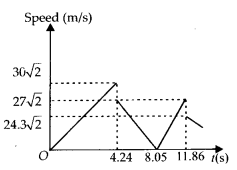Question 13.
Explain clearly, with examples, the distinction between:
(a) magnitude of displacement (sometimes called distance) over an interval of time, and the total length of path covered by a particle over the same interval.
(b) magnitude of average velocity over an interval of time, and the average speed over the same interval. [Average speed of a particle over an interval of time is defined as the total path length divided by the time interval]. Show in both
(a) and (b) that the second quantity is either greater than or equal to the first. When is the equality sign true? [For simplicity, consider one ­dimensional motion only].
(a) Magnitude of displacement of a particle in motion for a given time is the shortest distance between the initial and final positions of the particle in that time, whereas the total length of the path covered by particle is the actual path traversed by the particle in the given time. If a particle goes from A to B and B to C in time t as shown in figure, then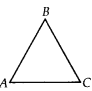Magnitude of displacement = distance AC. Total path length = distance AB + distance BC. From above we note that total path length (AB + BC) is greater than magnitude of displacement (AC).
If there is a motion of the particle in one dimension i.e. along a straight line, then the magnitude of displacement becomes equal to total path length traversed by the particle in the given time.

(b) Magnitude of average velocityAs, (AB + BC)> AC, so average speed is greater than the magnitude of average velocity. If the particle is moving along a straight line, then in a given time the magnitude of displacement is equal to total path length traversed by particle in that time, so average speed is equal to magnitude of average velocity.

Question 14.
A man walks on a straight road from his home to a market 2.5 km away with a speed of 5 km h-1. Finding the market closed, he instantly turns and walks back with a speed of 7.5 km h-1. What is the
(a) magnitude of average velocity, and
(b) average speed of the man over the interval of time (1) 0 to 30 min, (2) 0 to 50 min, (3) 0 to 40 min?
Here, distance of the market from the home of the man,
S = 2.5 km
Speed of the man, while going from his home to the market,
υ1 = 5 km h-1(1) Over the interval of time 0 to 30 min:
During this interval, the man covers distance from his home upto the market.
Therefore, displacement = 2.5 km; and
distance covered = 2.5 km
Now, time taken = 30 min = 0.5 h
Therefore, magnitude of the average velocity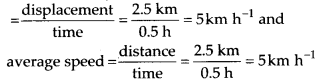(2) Over the interval of time 0 to 50 min:
During this time interval, the man returns his home.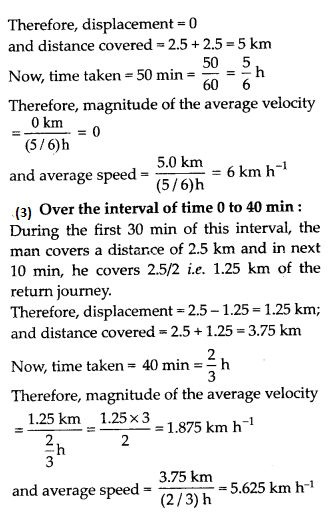Question 15.
In questions 13 and 14, we have carefully distinguished between average speed and magnitude of average velocity. No such distinction is necessary when we consider instantaneous speed and magnitude of velocity. The instantaneous speed is always equal to the magnitude of instantaneous velocity. Why?
The instantaneous velocity is defined as the velocity of an object at a particular instant of time.The instantaneous speed is defined as the limiting value of the average speed. Thus when time interval is very small, the magnitude of the displacement is effectively equal to the distance travelled by the object in the same small interval of time. Thus both instantaneous velocity and instantaneous speed are equal in this case. This can be understood from the following as :
Consider a small displacement over a time At between time interval, t and t + ΔtQuestion 16.
Look at the graphs (a) to (d) carefully and state, with reasons, which of these cannot possibly represent one-dimensional motion of a particle.(a) A line drawn for a given time parallel to position axis will cut the graph at two points which means that at a given instant of time, the particle will have two positions which is not possible. Hence graph (a) is not possible i.e. does not represent one dimensional motion.

(b) This graph does not represent one dimensional motion because at a given instant of time, the particle will have two values of velocity in positive, as well as in negative direction which is not possible in one dimensional motion.

(c)
This is a graph between speed and time and does not represent one dimensional motion as this graph tells that the particle can have the negative speed but the speed can never be negative.

(d)
This does not represent one dimensional motion, as this graph tells that the total path length decreases after certain time but total path length of a moving particle can never decrease with time.

Question 17.
Figure shows the (x-t) plot of one-dimensional motion of a particle. Is it correct to say from the graph that the particle moves in a straight line for t < 0 and on a parabolic path for t > 0? If not, suggest a suitable physical context for this graph.No, it is not correct to say that the particle moves in a straight line for t < 0 and on a parabolic path for t > 0 as the x-t graph does not show the trajectory of the path of a particle. The graph shows that at time t = 0, x = 0
Context : The suitable context is a body dropped from a tower i.e. free fall of a body i.e. x = 0 at t = 0

Question 18.
A police van moving on a highway with a speed of 30 km  h-1 fires a bullet at a thief’s car speeding away in the same direction with a speed of 192 km h-1. If the muzzle speed of the bullet is 150 m switch what speed does the bullet hit the thief’s car? (Note: Obtain that speed which is relevant for damaging the thief’s car).
Muzzle speed of bullet, υB = 150 m s -1 = 540 km h-1
Speed of police van,υP = 30 km h-1.
Speed of thief car, υT = 192 km h-1
Since the bullet is sharing the velocity of the police van, its effective velocity is
VB = υB + υP = 540 + 30 = 570 km h_1
The speed of the bullet w.r.t the thief’s car moving in the same direction
VBT = VB – υT = 570 -192 = 378 km h1

Question 19.
Suggest a suitable physical situation for each of the following graphs shown in figure.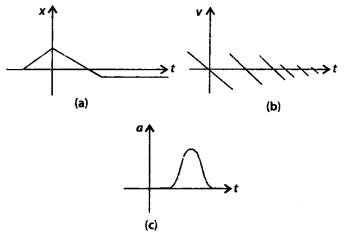Fig. (a) : The (x-t) graph shows that initially xis equal to 0, attains a certain value of x, again x becomes zero and then x increases in opposite direction till it again attains a constant x (i.e. comes to rest). Therefore, it may represent a physical situation such as a ball (initially at rest) on being kicked, rebounds from the wall with reduced speed and then moves to the opposite wall and then stops.

Fig. (b): From the (υ-t) graph, it follows that the velocity changes sign again and again with the passage of time and every time losing some speed. Therefore, it may represent a physical situation such as a ball falling freely (after being thrown up), on striking the ground rebounds with reduced speed after each hit against the ground.

Fig. (c) : The (a-t) graph shows that the body gets accelerated for a short duration only. Therefore, it may represent a physical situation such as a ball moving with uniform speed is hit with a bat for a very small time interval.

Question 20.
Figure gives the x-t plot of a particle executing one-dimensional simple harmonic motion. Give the signs of position, velocity and acceleration variables of the particle at t= 0.3 s, 1.2 s, -1.2 s.We know that in S.H.M., the acceleration ‘a’ is given by the relation,
a = -ω2x ………. (1)
where ω is the angular frequency and is a constant. Also velocity is given by,
υ=$\cfrac { dx}{dt}$  …………(2)

1. At time t = 0.3 s, x is negative, slope of (x-t) plot is negative, so position and velocity are negative but acceleration is positive according to equation (1).
2. At time, t = 1.2 s, x is positive, the slope of (x-t) plot is also positive, hence position and velocity are positive but according to equation (1), acceleration is negative.
3. At time, t = -1.2s, x is negative, the slope of (x-t) plot is also negative. But since both x and t are negative hence according to equation (2), velocity is positive. As position is negative, so according to equation (1) acceleration is positive.

Question 21.
Figure gives the (x-t) plot of a particle in one­ dimensional motion. Three different equal intervals of time are shown. In which interval is the average speed greatest, and in which is it the least? Give the sign of average velocity for each interval.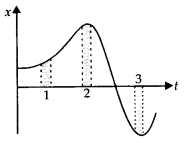We know that average speed in a small interval of time is equal to the slope of (x-t) graph in that interval of time. The average speed is the greatest in the interval 3 because slope is greatest and the average speed is least in interval 2 because slope is least there.The average speed is positive in intervals 1 and 2 because slope of (x-t) is positive there and average speed is negative in interval 3 because the slope of (x-t) is negative.

Question 22.
Figure gives a speed-time graph of a particle in one dimensional motion along a constant direction. Three equal intervals of time are shown. In which interval is the average acceleration greatest in magnitude? In which interval is the average speed greatest? Choosing the positive direction as the constant direction of motion, give the signs of v and a in the three intervals. What are the accelerations at the points A, B, C and D?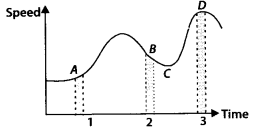(1) The magnitude of the average acceleration is given by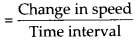i.e. average acceleration in a small interval of time is equal to the slope of (v-t) graph in that time interval.
As the slope of (υ-t) graph is maximum in the interval 2 as compared to intervals 1 and 3, hence the magnitude of average acceleration is greatest in interval 2.
(2) The average speed is greatest in the interval 3 as peak D is at maximum on speed axis.
(3) υ > 0 e. positive in all the three intervals.
(4) The slope is positive in intervals 1 and 3, so V e. acceleration is positive in these intervals while the slope is negative in interval 2, so acceleration is negative in it. So, a > 0 i.e. positive in intervals 1 and 3. and a < 0 i.e. negative in interval 2.
(5) As slope is zero at points A, B, C and D, so the acceleration is zero at all the four points.

Question 23.
A three-wheeler starts from rest, accelerates uniformly with 1 m s-2 on a straight road for 10 s, and then moves with uniform velocity. Plot the distance covered by the vehicle during the nth second (n = 1, 2, 3..) versus What do you expect this plot to be during accelerated motion: a straight line or a parabola?
Initial velocity, u = 0, a = 1 ms-2, t = 10 s, If S„ be the distance covered by the three­ wheeler in nth second. ThenThe following is the table for the distance Snth travelled in nth second.

 n(s) 1 2 3 4 5 6 7 8 9 10 v(m) 0.5 1.5 2.5 3.5 4.5 5.5 6.5 7.5 8.5 9.5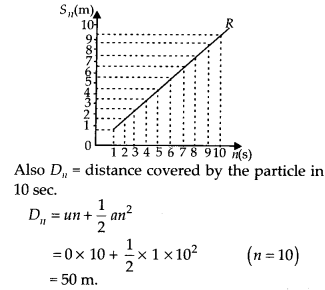As the equation describing the relation between D„ and n is of second degree, so the graph of the vehicle from start to 10th second is expected to be a parabola. Velocity of the vehicle at the end of 10th second is v = 0 + 1 x 10 = 10 m s’1 and it will move with this velocity after 10 seconds. Thus the graph will be a straight line inclined to time axis for uniformly accelerated motion. The plot of the distance covered by the three wheeler with time is shown in the graph.

Question 24.
A boy standing on a stationary lift (open from above) throws a ball upwards with the maximum initial speed he can, equal to 49 m s-1. How much time does the ball take to return to his hands? If the lift starts moving up with a uniform speed of 5 m s-1 and the boy again throws the ball up with the maximum speed he can, how long does the ball take to return to his hands?
Taking vertical upward direction as the positive direction of x-axis.
When lift is stationary, consider the motion of the ball going vertically upwards and coming down to the hands of the boy, we have u = 49 m s-1,
a = -9.8 m s-2, t = ?, x-x0 = S = 0
As, S = ut+$\cfrac {1}{2}$ at2
0 = 49t+$\cfrac {1}{2}$ (-9.8) t2

or  49f = 4.912 or f = 49/4.9 = 10 seconds As the lift starts moving upwards with uniform speed of 5 m s-1, there is no change in the relative velocity of the ball with respect to the boy i.e., it remains 49 m s’1. Hence, even in this case, the ball will return to the boy’s hand after 10 second.

Question 25.
On a long horizontally moving belt (shown in figure), a child runs to and fro with a speed 9 km h-1 (with respect to the belt) between his father and mother located 50 m apart on the moving belt. The belt moves with a speed of 4 km h-1. For an observer on a stationary platform outside, what is the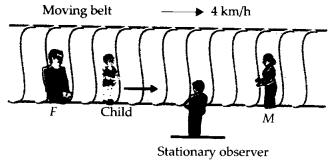(a) speed of the child running in the direction of motion of the belt?
(b) speed of the child running opposite to the direction of motion of the belt?
(c) time taken by the child in (a) and (b)?
Which of the answers alter if motion is viewed by one of the parents?
Let us consider left to right to be the positive direction of x-axis.
(a) Here, velocity of belt, υB = + 4 km h-1; Speed of child w.r.t. belt,
υc = + 9 km h-1 = 5/2 m s-1
Speed of the child w.r.t. stationary observer,
υc = υc +υB = 9 + 4 = 13 km h-1

(b)
Here, υB = +4 km h-1; υc = -9 km h-1
Speed of the child w.r.t. stationary observer,
υc– υc + υB = -9 + 4 = – 5 km h-1
Here negative sign shows that the child will appear to run in a direction opposite to the direction of motion of the belt.

(c)
Distance between the parents, S = 50 m. Since parents and child are located on the same belt, the speed of the child as observed by stationary observer in either direction (either from mother to father or from father to mother) will be 9 km
h-1.
Time taken by child in case (a) and (b) isIf motion is observed by one of the parents, answer to case (a) or case (b) will get altered. It is so because speed of child w.r.t. either mother or father is 9 km h1. But answer (c) remains unaltered due to the fact that parents and child are on the same belt and as such all are equally affected by the motion of the belt.

Question 26.
Two stones are thrown up simultaneously from the edge of a cliff 200 m high with initial speeds of
15 m s-1 and 30 m s-1. Verify that the graph shown in figure correctly represents the time variation of the relative position of the second stone with respect to the first. Neglect air resistance and assume that the stones do not rebound after hitting the ground.
Take g = 10 m s-2. Give the equations for the linear and curved parts of the plot.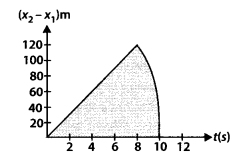Taking vertical upward motion of the first stone for time f, we have
x0 = 200 m, u = 15 m/s; a = -10 m/s2, t = t;x = x1
As, x = x0 +ut +$\cfrac {1}{2}$ at2
X1= 200 + 151 +$\cfrac {1}{2}$ (-10) t2
or X1 = 200 + 15 t -5 t2   ..(1)
Taking vertical upward motion of the second stone for time f, we have
x0 = 200 m, u = 30 m s_1, a = -10 m s-2, t=t,x= x1
Then x2 = 200 + 30t$\cfrac {1}{2}$ x 10t2
= 200+30 t-5t2    …(2)
When the first stone hits the ground, x1 = 0,
so t2 – 3t – 40 = 0
or (t – 8) (t + 5) = 0 Either t = 8 s or -5 s
Since t = 0 corresponds to the instant, when the stone was projected. Hence negative time has no meaning in this case.
So t = 8 s. When the second stone hits the ground, x2 = 0, so
0 = 200 + 30t – 5f2
or t2 -6t – 40 = 0
or (t – 10) (t+ 4) = 0
Therefore, either t = 10 s or t = -4 s
Since t = -4 s is meaningless, so t = 10 s Relative position of second stone w.r.t. first is
= x2-x1 = 15t    …(3)
From (1) and (2)
Since (x2 – x1) and t are linearly related, therefore, the graph is a straight line till t = 8 s. For maximum separation, t = 8 s, so maximum separation = 15 x 8 = 120 m after 8 second. Only second stone would be in motion for 2 seconds, so the graph is in accordance with the quadratic equation, x2 = 200 + 30t -5t2 for the interval of time 8 seconds to 10 seconds.

Question 27.
The speed-time graph of a particle moving along a fixed direction is shown in figure. Obtain the distance traversed by the particle between
(a) t = 0 s to 10 s,
(b) t = 2 s to 6 s.
What is the average speed of the particle over the intervals in (a) and (b)?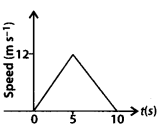(a) Let S be the distance covered by the particle between t = 0 to t = 10 s. Then,
S = area under (v-t) graph = area of the triangle, whose base is 10 (s) and height is 12 (m s-1)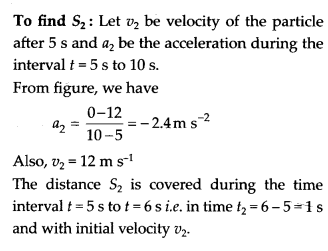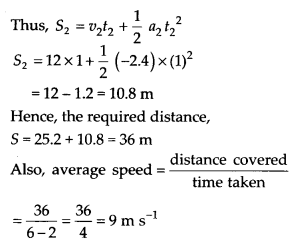Question 28.
The velocity-time graph of a particle in one­ dimensional motion is shown in figure. Which of the following formulae are correct for describing the motion of the particle over the time-interval tto t2:
(a) x(t2) = x(t1) + v(t1)(t2 – t1) + (1/2)a (t2 -t1)2
(b) v(t2) = v(t1) + a(t2-t1)
(c) vaverage = (x(t2) – x(t1))/(t2 -t1)
(d) average = M(t2) – v(t1))/(t2 – t1)
(e) x(t2) = x(t1) +vaverage(t2-t1)+ (1/2) oaverage (t2 – t1)2
(f) x(t2) – x(t1) = area under the v-t curve bounded by the t-axis and the dotted line shown.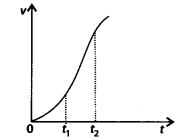(a) It is not correct. The reason is that in the time interval between t1 and t2, the velocity of the particle is not constant (slope of v-t graph is not same at all points).
(b) It is also not correct for the reason cited in (b)
(c)
It is correct.
(d) It is correct.
(e) It is not correct. In the expression, the average acceleration cannot be used.
(f) It is correct.

#### Plus One Physics Chapter Wise Textbook Solutions

Feel free to comment and share this article if you found it useful. Give your valuable suggestions in the comment session or contact us for any details regarding the HSE Kerala Plus One syllabus, Previous year question papers, and other study materials.